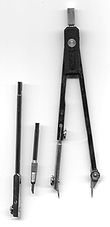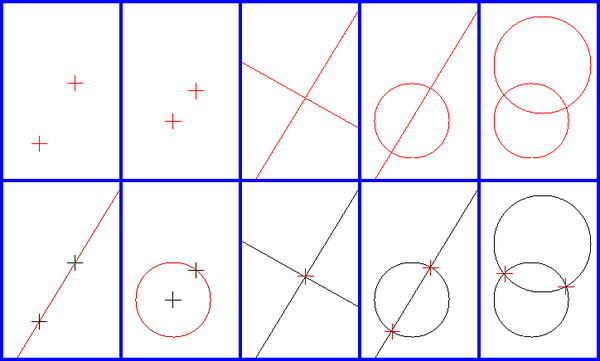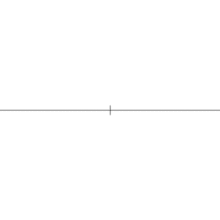# Compass and straightedge constructions

﻿
Compass and straightedge constructionsCreating a regular hexagon with a ruler and compassConstruction of a regular pentagon

Compass-and-straightedge or ruler-and-compass construction is the construction of lengths, angles, and other geometric figures using only an idealized ruler and compass.

The ruler to be used is assumed to be infinite in length, has no markings on it and only one edge, and is known as a straightedge. The compass is assumed to collapse when lifted from the page, so may not be directly used to transfer distances. (This is an unimportant restriction, as this may be achieved via the compass equivalence theorem.)

Every point constructible using straightedge and compass may be constructed using compass alone. A number of ancient problems in plane geometry impose this restriction.

The most famous ruler-and-compass problems have been proven impossible in several cases by Pierre Wantzel, using the mathematical theory of fields. In spite of existing proofs of impossibility, some persist in trying to solve these problems. Many of these problems are easily solvable provided that other geometric transformations are allowed: for example, doubling the cube is possible using geometric constructions, but not possible using ruler and compass alone.

Mathematician Underwood Dudley has made a sideline of collecting false ruler-and-compass proofs, as well as other work by mathematical cranks, and has collected them into several books.

## Compass and straightedge toolsA compass

The "compass" and "straightedge" of compass and straightedge constructions is an idealization of rulers and compasses in the real world:

• The compass can be opened arbitrarily wide, but (unlike some real compasses) it has no markings on it. It can only be opened to widths that have already been constructed, and it collapses when not used for drawing.
• The straightedge is infinitely long, but it has no markings on it and has only one edge, unlike ordinary rulers. It can only be used to draw a line segment between two points or to extend an existing line.

Each construction must be exact. "Eyeballing" it (essentially looking at the construction and guessing at its accuracy, or using some form of measurement, such as the units of measure on a ruler) and getting close does not count as a solution.

Stated this way, compass and straightedge constructions appear to be a parlour game, rather than a serious practical problem; but the purpose of the restriction is to ensure that constructions can be proven to be exactly correct, and is thus important to both drafting (design by both CAD software and traditional drafting with pencil, paper, straight-edge and compass) and the science of weights and measures, in which exact synthesis from reference bodies or materials is extremely important.[citation needed] One of the chief purposes of Greek mathematics was to find exact constructions for various lengths; for example, the side of a pentagon inscribed in a given circle. The Greeks did not find constructions for three problems:

For 2000 years people tried to find constructions within the limits set above, and failed. All three have now been proven under mathematical rules to be impossible generally (angles with certain values can be trisected, but not all possible angles.)

## The basic constructionsThe basic constructions

All compass and straightedge constructions consist of repeated application of five basic constructions using the points, lines and circles that have already been constructed. These are:

• Creating the line through two existing points
• Creating the circle through one point with centre another point
• Creating the point which is the intersection of two existing, non-parallel lines
• Creating the one or two points in the intersection of a line and a circle (if they intersect)
• Creating the one or two points in the intersection of two circles (if they intersect).

For example, starting with the minimal state of a drawing, with just two distinct points, we can create a line or either of two circles. From the two circles, two new points are created at their intersections. Drawing lines between the two original points and one of these new points completes the construction of an equilateral triangle.

Therefore, in any geometric problem we have an initial set of symbols (points and lines), an algorithm, and some results. From this perspective, geometry is equivalent to an axiomatic algebra, replacing its elements by symbols. Probably Gauss first realized this, and used it to prove the impossibility of some constructions; only much later did Hilbert find a complete set of axioms for geometry.

## Constructible points and lengthsTrisecting a segment with ruler and compass.

### Formal proof

There are many different ways to prove something is impossible. A more rigorous proof would be to demarcate the limit of the possible, and show that to solve these problems one must transgress that limit. Much of what can be constructed is covered in intercept theory.

We could associate an algebra to our geometry using a Cartesian coordinate system made of two lines, and represent points of our plane by vectors. Finally we can write these vectors as complex numbers.

Using the equations for lines and circles, one can show that the points at which they intersect lie in a quadratic extension of the smallest field F containing two points on the line, the center of the circle, and the radius of the circle. That is, they are of the form$x+y{\sqrt{k}}$, where x, y, and k are in F.

Since the field of constructible points is closed under square roots, it contains all points that can be obtained by a finite sequence of quadratic extensions of the field of complex numbers with rational coefficients. By the above paragraph, one can show that any constructible point can be obtained by such a sequence of extensions. As a corollary of this, one finds that the degree of the minimal polynomial for a constructible point (and therefore of any constructible length) is a power of 2. In particular, any constructible point (or length) is an algebraic number, though not every algebraic number (for example 32) is constructible (i.e. the relationship between constructible lengths and algebraic numbers is not bijective).

## Constructible angles

There is a bijection between the angles that are constructible and the points that are constructible on any constructible circle. The angles that are constructible form an abelian group under addition modulo 2π (which corresponds to multiplication of the points on the unit circle viewed as complex numbers). The angles that are constructible are exactly those whose tangent (or equivalently, sine or cosine) is constructible as a number. For example the regular heptadecagon is constructible because$\cos{\left(\frac{2\pi}{17}\right)} = -\frac{1}{16} \; + \; \frac{1}{16} \sqrt{17} \;+\; \frac{1}{16} \sqrt{34 - 2 \sqrt{17}} \;+\; \frac{1}{8} \sqrt{ 17 + 3 \sqrt{17} - \sqrt{34 - 2 \sqrt{17}} - 2 \sqrt{34 + 2 \sqrt{17}} }$

as discovered by Gauss.

The group of constructible angles is closed under the operation that halves angles (which corresponds to taking square roots). The only angles of finite order that may be constructed starting with two points are those whose order is either a power of two, or a product of a power of two and a set of distinct Fermat primes. In addition there is a dense set of constructible angles of infinite order.

## Compass and straightedge constructions as complex arithmetic

Given a set of points in the Euclidean plane, selecting any one of them to be called 0 and another to be called 1, together with an arbitrary choice of orientation allows us to consider the points as a set of complex numbers.

Given any such interpretation of a set of points as complex numbers, the points constructible using valid compass and straightedge constructions alone are precisely the elements of the smallest field containing the original set of points and closed under the complex conjugate and square root operations (to avoid ambiguity, we can specify the square root with complex argument less than π). The elements of this field are precisely those that may be expressed as a formula in the original points using only the operations of addition, subtraction, multiplication, division, complex conjugate, and square root, which is easily seen to be a countable dense subset of the plane. Each of these six operations corresponding to a simple compass and straightedge construction. From such a formula it is straightforward to produce a construction of the corresponding point by combining the constructions for each of the arithmetic operations. More efficient constructions of a particular set of points correspond to shortcuts in such calculations.

Equivalently (and with no need to arbitrarily choose two points) we can say that, given an arbitrary choice of orientation, a set of points determines a set of complex ratios given by the ratios of the differences between any two pairs of points. The set of ratios constructible using compass and straightedge from such a set of ratios is precisely the smallest field containing the original ratios and closed under taking complex conjugates and square roots.

For example the real part, imaginary part and modulus of a point or ratio z (taking one of the two viewpoints above) are constructible as these may be expressed as$\mathrm{Re}(z)=\frac{z+\bar z}{2}\;$$\mathrm{Im}(z)=\frac{z-\bar z}{2i}\;$$\left | z \right | = \sqrt{z \bar z}.\;$

Doubling the cube and trisection of an angle (except for special angles such as any φ such that φ/6π is a rational number with denominator the product of a power of two and a set of distinct Fermat primes) require ratios which are the solution to cubic equations, while squaring the circle requires a transcendental ratio. None of these are in the fields described, hence no compass and straightedge construction for these exists.

## Impossible constructions

### Squaring the circle

The most famous of these problems, squaring the circle, otherwise known as the quadrature of the circle, involves constructing a square with the same area as a given circle using only straightedge and compass.

Squaring the circle has been proven impossible, as it involves generating a transcendental ratio, namely$1/{\sqrt{\pi}}$. Only certain algebraic ratios can be constructed with ruler and compass alone, namely those constructed from the integers with a finite sequence of operations of addition, subtraction, multiplication, division, and square roots. The phrase "squaring the circle" is often used to mean "doing the impossible" for this reason.

Without the constraint of requiring solution by ruler and compass alone, the problem is easily solvable by a wide variety of geometric and algebraic means, and has been solved many times in antiquity.

### Doubling the cube

Doubling the cube: using only a straight-edge and compass, construct the side of a cube that has twice the volume of a cube with a given side. This is impossible because the cube root of 2, though algebraic, cannot be computed from integers by addition, subtraction, multiplication, division, and taking square roots. This follows because its minimal polynomial over the rationals has degree 3. This construction is possible using a ruler with two marks on it and a compass.

### Angle trisection

Angle trisection: using only a ruler and a compass, construct an angle that is one-third of a given arbitrary angle. This is impossible in the general case. For example: though the angle of π/3 radians (60°) cannot be trisected, the angle 2π/5 radians (72° = 360º/5) can be trisected.

## Constructing regular polygonsConstruction of a square.

Some regular polygons (e.g. a pentagon) are easy to construct with ruler and compass; others are not. This led to the question: Is it possible to construct all regular polygons with ruler and compass?

Carl Friedrich Gauss in 1796 showed that a regular n-sided polygon can be constructed with ruler and compass if the odd prime factors of n are distinct Fermat primes. Gauss conjectured that this condition was also necessary, but he offered no proof of this fact, which was provided by Pierre Wantzel in 1837.

## Constructing with only ruler or only compass

It is possible (according to the Mohr–Mascheroni theorem) to construct anything with just a compass if it can be constructed with a ruler and compass, provided that the given data and the data to be found consist of discrete points (not lines or circles). It is impossible to take a square root with just a ruler, so some things that cannot be constructed with a ruler can be constructed with a compass; but (by the Poncelet–Steiner theorem) given a single circle and its center, they can be constructed.

## Extended constructions

### Markable rulers

Archimedes and Apollonius gave constructions involving the use of a markable ruler. This would permit them, for example, to take a line segment, two lines (or circles), and a point; and then draw a line which passes through the given point and intersects both lines, and such that the distance between the points of intersection equals the given segment. This the Greeks called neusis ("inclination", "tendency" or "verging"), because the new line tends to the point. In this expanded scheme, any distance whose ratio to an existing distance is the solution of a cubic or a quartic equation is constructible. It follows that, if markable rulers and neusis are permitted, the trisection of the angle (see Archimedes' trisection) and the duplication of the cube can be achieved; the quadrature of the circle is still impossible. Some regular polygons, like the heptagon, become constructible; and John H. Conway gives constructions for several of them; but the 11-sided polygon, the hendecagon, is still impossible, and infinitely many others.

When only an angle trisector is permitted, there is a complete description of all regular polygons which can be constructed, including above mentioned regular heptagon, triskaidecagon (13-gon) and enneadecagon (19-gon). It is open whether there are infinitely many primes p for which a regular p-gon is constructible with ruler, compass and an angle trisector.

### Origami

Similarly, the mathematical theory of origami (i.e. paper folding without any equipment) is more powerful than the compass and staightedge, for the same reasons. It can also be used to solve cubic equations (and hence quartic equations), and thus solve two of the classical problems .

Origami can construct exactly the same set of points as the extended constructions using a compass and a marked ruler.

### The extension field

In abstract terms, using these more powerful tools of either neusis using a markable ruler or the constructions of origami extends the field of constructible numbers to a larger subfield of the complex numbers, which contains not only the square root, but also the cube roots, of every element. The arithmetic formulae for constructible points described above have analogies in this larger field, allowing formulae that include cube roots as well. The field extension generated by any additional point constructible in this larger field has degree a multiple of a power of two and a power of three, and may be broken into a tower of extensions of degree 2 and 3.

## Recent research

Simon Plouffe has written a paper showing how ruler and compass can be used as a simple computer with unexpected power to compute binary digits of certain numbers.

Wikimedia Foundation. 2010.

### Look at other dictionaries:

• Compass equivalence theorem — The compass equivalence theorem is an important statement in compass and straightedge constructions. In these constructions it is assumed that whenever a compass is lifted from a page, it collapses, so that it may not be directly used to transfer …   Wikipedia

• Straightedge — A straightedge is a tool with an accurately straight edge used for drawing or cutting straight lines, or checking the straightness of lines. If it has equally spaced markings along its length it is usually called a ruler.True straightness can in… …   Wikipedia

• Constructible polygon — Construction of a regular pentagon In mathematics, a constructible polygon is a regular polygon that can be constructed with compass and straightedge. For example, a regular pentagon is constructible with compass and straightedge while a regular… …   Wikipedia

• Huzita–Hatori axioms — The Huzita–Hatori axioms or Huzita–Justin axioms are a set of rules related to the mathematical principles of paper folding, describing the operations that can be made when folding a piece of paper. The axioms assume that the operations are… …   Wikipedia

• Euclidean geometry — A Greek mathematician performing a geometric construction with a compass, from The School of Athens by Raphael. Euclidean geometry is a mathematical system attributed to the Alexandrian Greek mathematician Euclid, which he described in his… …   Wikipedia

• Angle trisection — The problem of trisecting the angle is a classic problem of compass and straightedge constructions of ancient Greek mathematics.Two tools are allowed # An un marked straightedge, and # a compass, Problem: construct an angle one third a given… …   Wikipedia

• Problem of Apollonius — In Euclidean plane geometry, Apollonius problem is to construct circles that are tangent to three given circles in a plane (Figure 1); two circles are tangent if they touch at a single point. Apollonius of Perga (ca. 262 BC ndash; ca. 190 BC)… …   Wikipedia

• Émile Lemoine — Infobox Scientist name = Émile Michel Hyacinthe Lemoine image width = 250px caption = birth date = birth date|1840|11|22|fd=y birth place = Quimper, France residence = Paris, France nationality = French death date = death date and… …   Wikipedia

• Special cases of Apollonius' problem — In Euclidean geometry, Apollonius problem is to construct all the circles that are tangent to three given circles. Limiting cases of Apollonius problem are those in which at least one of the given circles is a point or line, i.e., is a circle of… …   Wikipedia

• History of geometry — Geometry (Greek γεωμετρία ; geo = earth, metria = measure) arose as the field of knowledge dealing with spatial relationships. Geometry was one of the two fields of pre modern mathematics, the other being the study of numbers. Classic geometry… …   Wikipedia